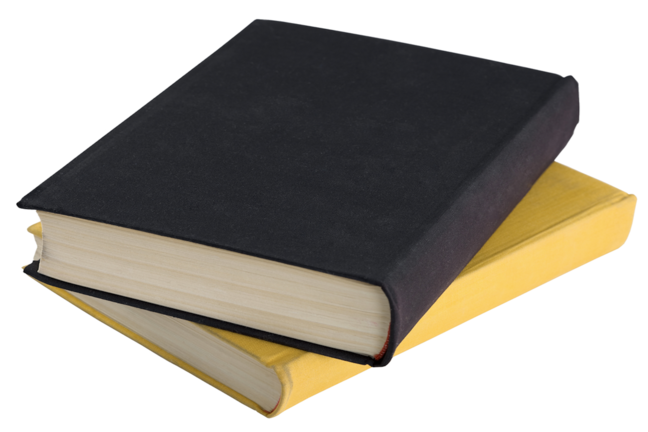2.21

## Weizmann Institute of Science# ISBNs and checksum digits

Before we take a look at checksums, I’d like to backtrack a bit and look at a special kind of serial number: ISBNs (International Standard Book Number).

## ISBNs

Books can be arranged in many different ways. We can arrange them by their subject, by the name of author, or according to the book’s title. In the past, librarians used to write down the location of each book on index cards. Librarians had to be extremely well-organised, otherwise the books would get lost. Fortunately, nowadays, the books are registered on a computer. Details such as the name of the author, the publisher, number of pages, and location inside the library are recorded next to the book’s title.

To make matters easier for the computer system (and us), each book is assigned a serial number, the ISBN, which serves as its “ID code”. Take the paperback: “Harry Potter and the Philosopher’s Stone” and look at the first page. Our English edition of the book has the following identification number written on the first page: ISBN 0747532745 .
The ISBN also appears on the back, usually just above the barcode…”. It is an international identification code, unique to each edition of the book and is usually published on the book’s back cover, as well as on the copyright page (one of the first pages of the book). Until the end of 2006 the ISBN had ten digits, and was called ISBN-10. As of January 1st, 2007, it has thirteen digits, and is called ISBN-13.

## ISBN-10 and check digits

Let’s take a look at the structure of ISBN-10.

The ten digits of ISBN-10 are divided into four parts, sometimes separated by a hyphen (-), the four parts are:

• A number for the language of the book (for example, 0 represents books in English, 2 represents books in French, 88 - Italian, 965 - Hebrew etc.)
• A number representing the book’s publisher
• The book’s serial number (used by the publisher)
• The last digit – the rightmost digit - is called the check digit.

There are many examples of places that use check digits. One example is the last digit of a passport number. Usually, it is used when there are large numbers that have many digits. When copying such a number, there is a greater chance of making a mistake in one of the digits. The purpose of the check digit is to find these mistakes.

Nowadays, computers are used to calculate the check digit. When planning the check digit calculation we try to make it so that many mistakes as possible are discovered. In the 1950s, the German American mathematician Hans Peter Luhn developed an algorithm for calculating check digits that is used all over the world to this day.

The ISBN check digit is chosen so that a mathematical manipulation on all the ten digits of the number will always result in a number that is divisible by 11 without remainder. Once the digit is chosen and we have the full ISBN number, we can check if it is valid using the following algorithm:

1. Multiply the first digit by 1, the second by 2, the third by 3, and so on until you reach the last digit, which should be multiplied by 10.
2. Add up the ten numbers (products) you got in the previous step, and divide this sum by 11 (you can use a calculator) and take a look at the final result:
• If it is a whole number (meaning there was no remainder or fraction), then the ISBN is valid, and you’re done!
• Otherwise, the ISBN is not valid… ☹

Example: the ISBN 965-448-765-9 (of the Hebrew translation of ‘Harry Potter and the Philosopher’s Stone’)

1. Multiply the ISBN’s digits by 1 to 10:
9×1 + 6×2 + 5×3 + 4×4 + 4×5 + 8×6 + 7×7 + 6×8 + 5×9 + 9×10
2. Add up the ten numbers (products) from the previous step:
9 + 12 + 15 + 16 + 20 + 48 + 49 + 48 + 45 + 90 = 352
3. Divide the sum by 11:
352 ÷ 11 = 32
The result is a whole number, and there is no remainder → the ISBN is valid!

## Checksums in barcodes

The rightmost digit is a check digit in most of the barcodes also. This digit is also known as the checksum. Let’s take a look at checksums in another common barcode - EAN-13. We will not go into the details of its structure here, and mention only that if the first digit in this code is 0, then the code is identical to UPC-12, which we have already met.
The rightmost digit in products of this type is a check digit, which is calculated in the following way:

• The first digit in the barcode is multiplied by 1, the second by 3, the third by 1 again, the fourth by 3 and so on, so that the digits are multiplied alternately by 1 and 3
• The products are added up
• The check digit (which is the 13th digit) should be the difference between the sum from the second step and the closest multiple of 10 that is larger or equal to this sum.

For example, let’s check: the code 729000017472? (? represents the check digit).
We perform the multiplication and summing according to the instructions:
7×1+2×3+9×1+0×3+0×1+0×3+0×1+1×3+7×1+4×3+7×1+2×3=57
The closest multiple of 10 greater than 57 is 60. The difference between 60 and 57 is 3, so the check digit is 3.

Now we know all that we need to take a broader look at barcodes, before we challenge you to read some with your naked eyes…